Support the Monkey! Tell All your Friends and TeachersHome MonkeyNotes Printable Notes Digital Library Study Guides Study Smart Parents Tips College Planning Test Prep Fun Zone Help / FAQ How to Cite New Title Request

 7.2 Algebra of Vectors (1) Addition of two Vectors Triangle Law :- The sum or resultant of two vectors a and b is the vector formed by placing the initial point of b on the terminal point of a and then joining the initial point of a to the terminal point of b . This sum is written as r = a + b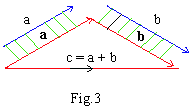This law is also known as Tail - Tip rule Parallelogram Law :- It states that the sum or resultant of a and b is R is the diagonal of the parallelogram for which a and b are adjacent sides. All vectors a, b and R are concurrent as shown in figure 4.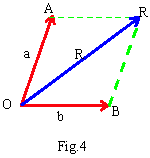Vector addition is commutative i.e. a + b = b+ c Extension to sums of two or more vectors is immediate. Vector addition is associative. Observe the adjoining fig.5 we have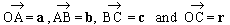using tail-tip rule, for triangle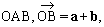for triangle ABC,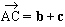for triangle OBC, c i.e. r = ( a + b) + c . Also, for triangle OAC ,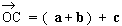i.e r = a + (b + c). Thus , r = ( a + b ) + c = a + (b + c).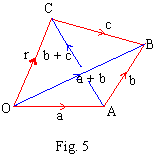2) Subtraction of a Vector :- It is accomplished by adding the negative of vectors i.e. a - b = a + ( - b). Note also that - ( a + b ) = - a - b . 3) Composition of Vectors :- It is the process of determining the resultant of a system of vectors. For this we have to draw a vector polygon, placing tail of each vector at the tip of the preceding vector. Then draw a vector from the tail of the first vector to tip of the last vector in the system. Later on we will show that not all vector systems reduce to a single vector. Also note that the order of vectors are drawn is immaterial.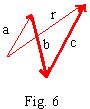Free Vector :- A vector which can be shifted along its line of action or parallel to itself and whose initial point can be anywhere, is called a free vector. Unless stated, we always refer a vector as a free vector . Localized Vector :- It is also known as a sliding vector. It can be shifted only along its line of action. By the principle of transmissibility the external effects of this vector remains the same. Bounded Vector :- It is called as a fixed vector. It must remain at the same point of action or application. Negative of a Vector :- The negative of a vector u is the vector -u which has the same direction and inclination but is of the opposite sense (of direction). * The resultant of a system of vectors is the least number of vectors that will replace the given system. Position Vector of a Point :- If A and B are any two points then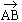is called the position vector of point B with reference to point A.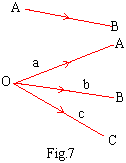Usually we fix a point O and define the position vector of points with reference to O which is called the origin of reference. We shall use a, b, c etc. to denote position vectors of points A, B, C etc. respectively, relative to origin O. Unit Vector :- A vector in a given direction having unit magnitude is called a unit vector in that direction . It is usually denoted by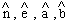etc ( Read as n Cap). Also, note that if U is given vector then the unit vector in the direction (or along or parallel ) to u is given by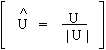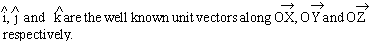Index Chapter 8
 Search: All Products Books Popular Music Classical Music Video DVD Toys & Games Electronics Software Tools & Hardware Outdoor Living Kitchen & Housewares Camera & Photo Cell Phones Keywords: